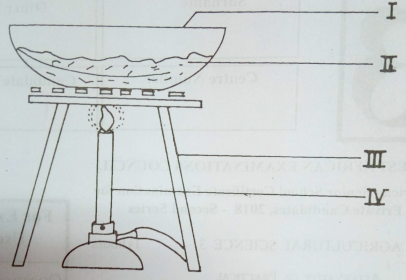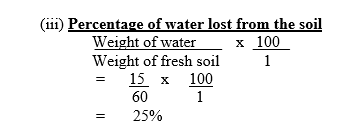Question 1

• 1. The diagram below illustrates an experimental set-up in soil analysis. Study it carefully and answer the questions that follow.(a) State the aim of the illustrated experiment.  (1 mark)

(b) Name the part labelled I, II, III and IV.   (4 marks)

(c)  A fresh sample of soil weighed 60 g. A container weighing 35 g was used to dry the soil. If both the soil and the container weighed 80 g after the experiment, determine the:
(i) weight of the dried soil;  (2 marks)
(ii) weight of the water lost from the soil;   (2 marks)
(iii) percentage of water lost from the soil.   (3 marks)

(d) List three forms in which water exists in the soil. (3 marks)

Observation

•

This question was not popular among candidates.
(a) Many candidates were unable to state the aim of the experiment as well as name the illustrated parts in the experimental set-up.

(b) Candidates performed poorly in the calculations on soil analysis.

(c) Most candidates were however able to mention the forms in which water exists in the soil.

(a)  Aim of the illustrated experiment

Determination of moisture content of a soil sample or organic matter content of the soil.

(b)  Labelled parts in the illustrated experiment
I   -  Evaporating dish/ crucible
II  -  Soil
III - Tripod stand
IV - Bunsen burner
(c) (i)  Weight of dried soil
Weight of empty evaporating dish  =  35g
Weight of dish  +  dried soil  =  80g
Weight of dried soil only  =  (80 - 35)g
=  45g

(ii) Weight of water lost from the soil
(60 - 45)g
=  15g
OR
(95 - 80)g
=  15g
(iii) Percentage of water lost from the soil(d)  Forms in which water exists in the soil

• Hygroscopic water
• Capillary water
• Gravitational water